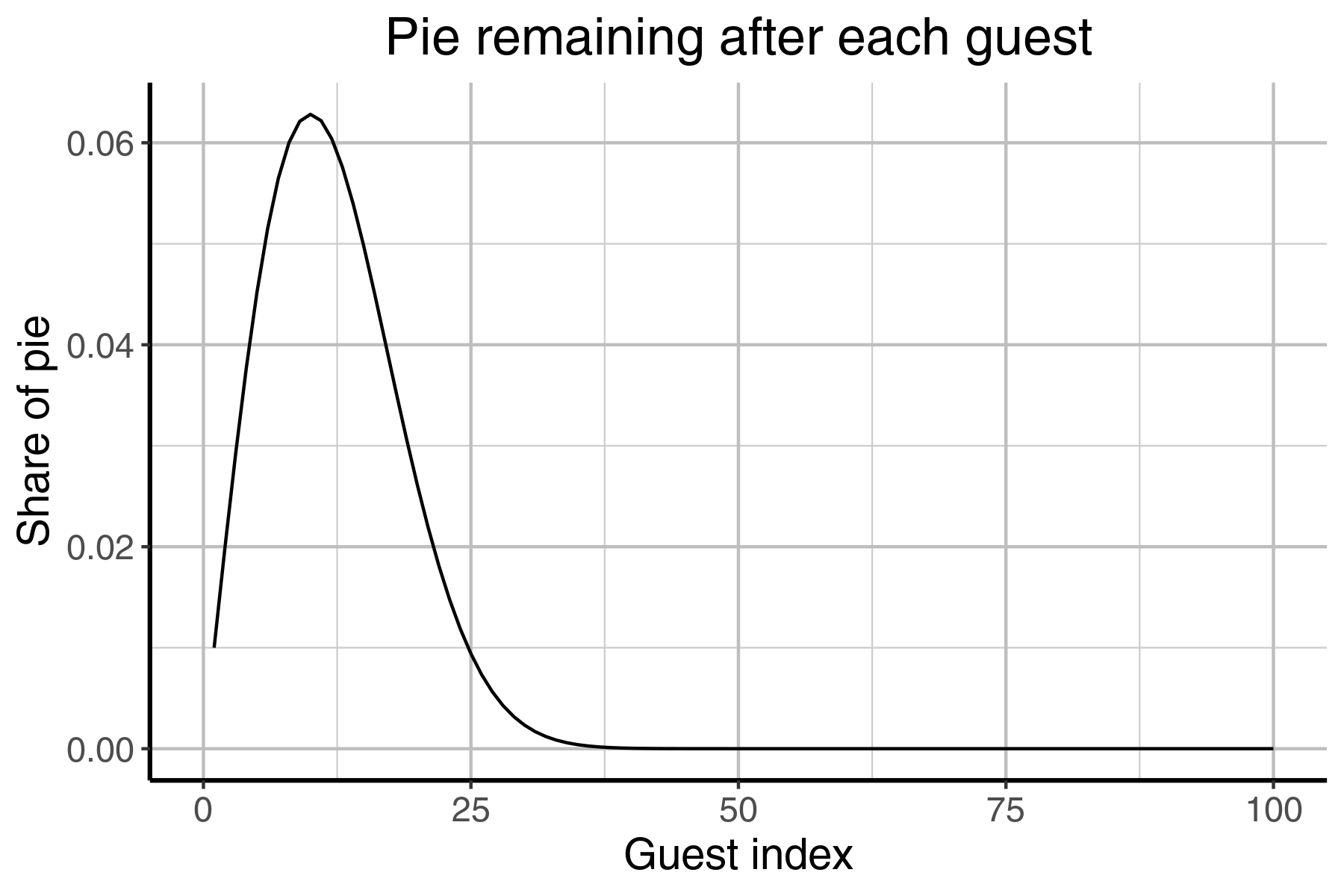This week’s Perl Weekly Challenge includes a math puzzle known as the Pythagoreas Pie Puzzle. The problem, as stated by challenge proponent Jo Christian Oterhals:

At a party a pie is to be shared by 100 guest. The first guest gets 1% of the pie, the second guest gets 2% of the remaining pie, the third gets 3% of the remaining pie, the fourth gets 4% and so on.

Write a script that figures out which guest gets the largest piece of pie.

Let’s take a look at what happens for the first few guests when time they come to take a slice of the pie. The table below shows the calculation made for each guest:

Guest (index) Portion
1 (0.01*100)
2 (0.02*99)
3 (0.03*97.02)

There’s clearly a pattern there:

• Each guest is getting a fixed percentage of the remaining pie
• The fixed percentage is dependent on the guest’s “index”
• The calculation of that depends on the index of the guest and the remaining pie when they came along.

So each guest has to do something like:

$$(i/100)R$$

where $$i$$ is the index of the guest and $$R$$ is how much pie is remaining, to calculate how much they get. Given the table and the points noted above, it becomes clear that the way we get $$R$$ is just by going back to the calculation made by the guest before, all the way back to the first one:

\begin{align} 100 \times \frac{99}{100} & \quad \text{First guest} \newline 100 \times \frac{99}{100} \times \frac{98}{100} & \quad \text{Second guest} \newline 100 \times \frac{99}{100} \times \frac{98}{100} \times \frac{97}{100} & \quad \text{Third guest} \newline \vdots \newline \end{align}

Why $$\frac{99}{100}$$ and not $$\frac{1}{100}$$, and so on, in the equations above? Because the next guest needs to know how much of the pie is left, not how much the guest before them took. The first guest left behind (99100) of 100% of the pie. The next guest left behind 98100 of that. And so on.

This problem can be considered as a product of a sequence, with a general formula of

$$\prod_{i=1}^{99} {\frac{(100-i)}{100}}$$

We can start at $$i=1$$ and not $$i=0$$ because that is just a multiplication by 1. We also don’t go to 100 since after the last guest nothing is left ($$\frac{100-100}{100} = 0$$). If the upper limit were 3, we would get the amount of pie remaining after 3 guests

\begin{align} \prod_{i=1}^{3} {\frac{(100-i)}{100}} &= \frac{99}{100} \times \frac{98}{100} \times \frac{97}{100}\newline &= 0.941094\newline \end{align}

The first iteration of my code used this observation, keeping a list of the amount of pie left after each guest. The next value, $$i$$, in the list is calculated simply by multiplying the last element in the array by $$\frac{100-i}{100}$$ since that value is already the product of all prior multiplications up to that point. Then I would take the difference between the sizes of the remaining pie after the current guest and the guest before:

sub list_pie_remaining {

# Other guests get a percent of the remaining 99/100:
# 99/100*(2/100) for the second, leaving (99/100)*(98/100)
# for the third guest, and so on...
# So lets just generate a list with the amount of pie left
# for each guest
my @remaining_pie = (1);

# my @pie_shares = (1/100);
my %largest_piece = ( guest => -1, size => 0 );

for my $i ( 1 .. 99 ) { push @remaining_pie, ( ( 100 -$i ) / 100 ) * $remaining_pie[-1]; ( ($remaining_pie[-2] - $remaining_pie[-1] ) >$largest_piece{size} )
? @largest_piece{ "guest", "size" }
= ( $i,$remaining_pie[-2] - $remaining_pie[-1] ) : next; # push @pie_pieces,$remaining_pie[-2]-$remaining_pie[-1]; } return \%largest_piece; } I kept a hash with the largest piece seen so far, which is returned as a hashref. It turns out, however, that the shares each guest gets are actually getting larger right up until a certain point, as shown in the graph below:Which means that we only really need to keep track of values for the pie share right up until the share sizes start to decrease, halting execution much sooner than in my first try at this. Late spoiler alert: it helps if you already knew the answer ahead of time, but the largest slice is taken by the 10th guest, so we save needless calculations over the next 90 guests! Another change we could make is that we don’t need to maintin a list of all prior values. You could just keep track of the last piece and the pie remaining: sub track_last_piece { my$remaining_pie = 1;
my $last_piece = 0; for my$i ( 1 .. 100 ) {
my $new_piece =$remaining_pie - ( ( 100 - $i ) / 100 ) *$remaining_pie;

if ( $new_piece <$last_piece ) {
return { guest => ( $i - 1 ), size =>$last_piece };
}

$last_piece =$new_piece;
$remaining_pie -=$new_piece;
}

return undef;
}

Obviously, I won’t claim that this is the best solution, but the best I came up with, in the time I had. I took a page out of Steven Wilson’s book and used Benchmark::Forking as he details in his awesome blog post on last week’s challenge. Below are the results I got when comparing the two methods:

                        Rate list_pie_remaining   track_last_share
list_pie_remaining  43203/s                 --               -88%
track_last_piece   368816/s               754%                 --

Obviously a dramatic improvement, which almost certainly leaves room for improvement.

See the full solution, here.